Blog

5000+ Members

MEETUPS

LEARN, CONNECT, SHARE

JOB POSTINGS

INDEED POSTINGS

Browse through the latest deep learning, ai, machine learning postings from Indeed for the GTA.

CONTACT

CONNECT WITH US

Are you looking to sponsor space, be a speaker, or volunteer, feel free to give us a shout.

Sample Efficient Evolutionary Algorithm for Analog Circuit Design

In this post, we share some recent promising results regarding the applications
of Deep Learning in analog IC design. While this work targets a specific
application, the proposed methods can be used in other black box optimization
problems where the environment lacks a cheap/fast evaluation procedure.

<!–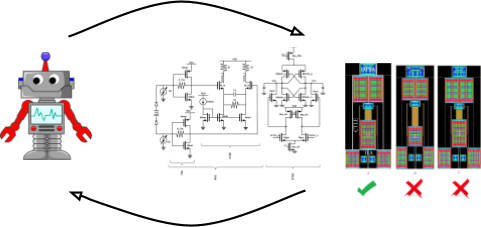–>So let’s break down how the analog IC design process is usually done, and then
how we incorporated deep learning to ease the flow.

The intent of analog IC design is to build a physical manufacturable circuit
that processes electrical signals in the analog domain, despite all sorts of
noise sources that may affect the fidelity of signals. Usually analog circuit
design starts off with topology selection. Generically speaking, engineers
usually come up with topology of certain blocks and try to size them such that
after putting them together the entire system behaves in a certain way and
satisfies some figures of merit. There are certain levels of simulations and
tests that need to take place to verify that the system will work before
manufacturing. At the lowest level engineers do their design using their
intuition and equations and then simulate and make the corresponding changes
until they converge to a working design. The more accurate the simulation, the
more time it takes to run. Unfortunately, in recent advanced technologies the
large disparity between post-layout simulation (physical realization of the
circuit) and schematic simulation (circuit concept) requires designers to be
aware of the parasitic effects due to the way the circuit is physically
implemented. This basically means that simulations take longer, and on top of
that more manual iterations are needed.

Now that we understand the environment let’s give a brief overview of past
attempts to automate some parts of this process.

Historically people have tried different degrees of automation in different
parts of the design flow (a complete ancient survey can be found in this
textbook
, and more recent work includes Bayesian optimization and
RL). We are mostly interested in approaches that find the optimal sizing for a
given topology to satisfy a collection of metrics (a constraint satisfaction
problem).

Some people have derived analytical formulations for behavior of circuits and turned the problem into optimization over these analytical expressions (for example by expressing gain as an analytical function of transistor sizes). However, as was mentioned earlier, today, even schematic simulations can differ from their layout counterpart. So those ancient approaches lost their attractiveness very early on due to this important
drawback.

Some other approaches tried to use simulations and modeled the problem as a
black box optimization. A lot of them showed success in simple circuits in
schematic-based simulations, but again could not scale very well to larger
circuits and layout exploration. The bottom line till now is that a lot of
iterations are needed and long simulations make it even more difficult and
cumbersome.

On a side note, population based black-box optimization algorithms achieve a pretty good performance, in terms of the final output’s quality.
For example, this paper shows a setting where RL agents are trained in a parallelized fashion using scalable evolutionary algorithms. The problem is that they are insanely sample inefficient (despite
being parallelizable) and their exploration strategy is mostly stochastic with
no “real” guidance. The problem with circuit design is that tools available for
simulation are not highly parallelizable or very expensive to parallelize. In this work we have proposed a new way to make them more sample efficient.

Let’s formalize the problem a bit. Let’s say we want to design an amplifier
with a given topology for a gain larger than \$A_0\$ and a bandwidth larger than
\$BW_0\$. We want to find the “optimum” sizes for the components of the circuit such that they satisfy these performance constraints.
We can formulate the problem as minimizing a scalar cost function equal to the sum of relative errors to the required specification. More concretely, in this example:

% <![CDATA[
cost(x) = frac{|A(x) – A_0|}{(A(x) + A_0)} mathbf{1}(A(x) < A_0) +
frac{|BW(x) – BW_0|}{(BW(x) + BW_0)} mathbf{1}({BW(x)

And we get the \$A(x)\$ and \$BW(x)\$ values after simulation.

To state it in a more general form:

cost(x) = sum_{i}{w_ip_i(x)}

Where \$x\$ presents the geometric parameters in the circuit topology and

p_i(x)= frac{|c_i – c_i^*|}{c_i+c_i^*}

represents the normalized spec error for designs that do not satisfy constraint
c_i^*, or zero if they do. \$c_i\$ denotes the value of constraint \$i\$ at
input \$x\$, and is evaluated using a simulation framework. c_i^* denotes the
optimal value. Intuitively this cost function is only accounting for the
normalized error from the unsatisfied constraints, and \$w_i\$ is the tuning
factor, determined by the designer, which controls prioritizing one metric over
another if the design is infeasible.

In principle, we can start optimizing \$cost(x)\$ by using evolutionary
algorithms (a great intro found here). In fact we used deap to
implement a baseline version of genetic algorithm to our problem. The issue is that
there is no “real” intelligence in exploration, and therefore, it takes a lot of
expensive simulations to find a solution, even for the simplest design
problems. To put it in perspective let’s work with a 3D contrived example,
let’s say the current population includes \$x_1\$, \$x_2\$, and \$x_3\$ among which
none satisfy all specs under consideration.

<!–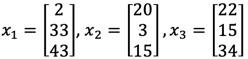–>

begin{align*}
x_1 = begin{bmatrix}
2 \
33 \
43
end{bmatrix},
x_2 = begin{bmatrix}
20 \
3 \
15
end{bmatrix},
x_3 =
begin{bmatrix}
22 \
15 \
34
end{bmatrix},
end{align*}

Now let’s say our chance hits and we produce the following \$y\$ samples from the
old population.

<!–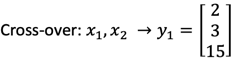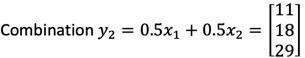–>

begin{align*}
text{cross-over}: x_1, x_2 to y_1 = begin{bmatrix}
2 \
3 \
15
end{bmatrix}
end{align*}begin{align*}
text{combination}: y_2 = 0.5x_1 + 0.5x_2 = begin{bmatrix}
11 \
18 \
29
end{bmatrix}
end{align*}begin{align*}
text{Mutation}: y_3 = text{Mutate}(x_3)= begin{bmatrix}
50 \
15 \
10
end{bmatrix}
end{align*}

We know the performance of \$x\$ samples and to know performance of \$y\$ samples
we need to run simulations (this is the part which can potentially be extremely
slow).

Now let’s say after simulation we sort samples by their performance and get the
following next generation of population.

<!––>

begin{align*}
begin{bmatrix}
x_1 \
y_1 \
x_2 \
x_3 \
y_2 \
y_3 \
end{bmatrix} to
begin{bmatrix}
x_1 \
y_1 \
x_2 \
end{bmatrix}
end{align*}

Observe that after this “unlucky” iteration \$y_2\$ and \$y_3\$ got eliminated. In
our proposed method, we devised a model to predict the performance before
simulation and only simulate those samples which have better predicted
performance. So in our contrived example we’ll predict whether new designs have
better performance than \$x_1\$ and if so, we simulate them. If our prediction is
accurate (or almost accurate) we waste fewer simulations. On the other hand,
if we do not make accurate decisions, we could either approve samples that
do not show high quality after simulation or reject designs that
should have been accepted.

Next we’ll describe a model that was able to achieve acceptable results
utilizing this idea. All implementations details can be found in the GitHub
code
.

Architecture Choice for the Model

This model has to have two distinct characteristics:

1. It has to be able to express how good/bad a new, not simulated sample is
compared to individuals in the current population.

2. It should be able to generalize well, given very limited number of training
samples (potentially 100-300 accurate simulations). However, it should not be
biased towards a specific region in space and get stuck in a local optimum.

The first potential candidate is a regression model which predicts the cost
value and uses that prediction to determine whether to simulate a design. The
cost function that the network tries to approximate can be a non-convex and
ill-conditioned one. Thus, from a limited number of samples it is very unlikely
that it would generalize well to unseen data. Moreover, the cost function
captures too much information from a single scalar number, so it would be hard
to train, given a small number of training points and then expect it to
generalize well.

Another option is to predict the value of each metric (i.e. gain, bandwidth,
etc.). While the individual metric behaviour can be smoother than the cost
function, predicting the actual metric value is unnecessary, since we are
simply attempting to predict whether a new design is superior to some other
design. Therefore, instead of predicting metric values exactly, the model can
take two designs and predict only which design performs better in each
individual metric.

We know that there are certain patterns in circuit parameters that make some
metrics better than others (for example upsizing all transistors make the
amplifier faster but costs more power). We use those parameters as the input of
our network. Moreover, by forming a model that takes in pairs of designs
instead of a single design we effectively expand our training sample size (a
population of size 100 has ~5000 pairs). Despite the fact that neural net’s
input space is larger, in practice, training is easier.

The bottom figure shows the structure of the neural net modeling the oracle
simulator used in this approach. This model takes in two sets
of parameters (one for Design A and one for B); extracts some useful features
\$(f_1, ldots, f_k)\$; rearranges the order of features (the reason for this
will become clear shortly); and feeds the cascaded/rearranged feature vectors
to independent neural networks to predict the superiority of Design A to Design
B for a given spec. The dedicated superiority-predicting neural nets share the
same architecture across all specs, but are parameterized separately. The
output is interpreted as the probability that Design A is better than Design B
in \$text{spec}_1\$ or \$text{spec}_2\$ and so on.

So during inference, the parameters of the query design are going to be fed to
Design B, and the reference design to Design A. Then we predict how the query
design will do compared to the reference design. Then we use some heuristic to
decide whether to simulate or ignore the new design.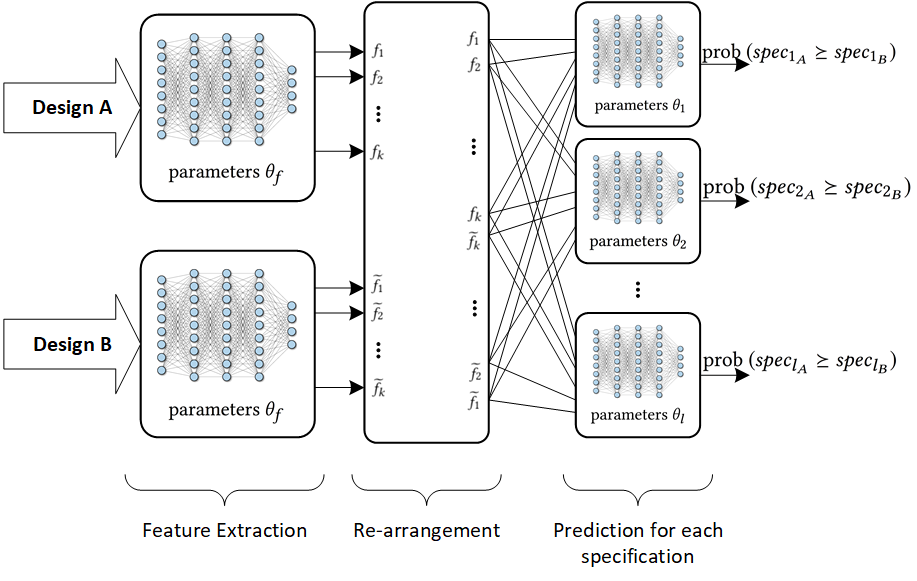One other subtle constraint on the model is that there should be no
contradiction in the predicted probabilities depending on the order by which
the inputs were fed in. For example if we feed in A and B, and A is predicted
to have a better gain than B with probability 0.8, if we swap the order of A
and B the probability should become 0.2 by construction of the network.
This requires a particular symmetry in the network architecture. For
more info on this refer to the paper, but here is a code snippet that
shows how we make sure a layer behaves like that by construction.

weight_elements = tf.get_variable(name='W', shape=[input_data.shape//2, layer_dim], initializer=tf.random_normal_initializer)
bias_elements = tf.get_variable(name='b', shape=[layer_dim//2], initializer=tf.zeros_initializer)
Weight = tf.concat([weight_elements, weight_elements[::-1, ::-1]], axis=0, name='Weights')
Bias = tf.concat([bias_elements, bias_elements[::-1]], axis=0, name='Bias')

The reason that the rearrangement happens is exactly this and the code below shows
how it’s actually done.

features1 = self._feature_extraction_model(input1_norm, name='feat_model', reuse=False)
features2 = self._feature_extraction_model(input2_norm, name='feat_model', reuse=True)
input_features = tf.concat([features1, features2[:, ::-1]], axis=1)

To train the network, we construct all Design A and Design B permutations from
the buffer of previously simulated designs and label their comparison in each
metric. We then update network parameters with Adam optimizer, using sum of
cross-entropy loss for all metrics.

There is also another architecture choice that helped in the overall
convergence and that is using dropout layers to estimate the uncertainty in
predictions. During each query, we randomly turn off 20% of the activations in
each layer 5 times, and average the output probabilities to remove the
uncertainty.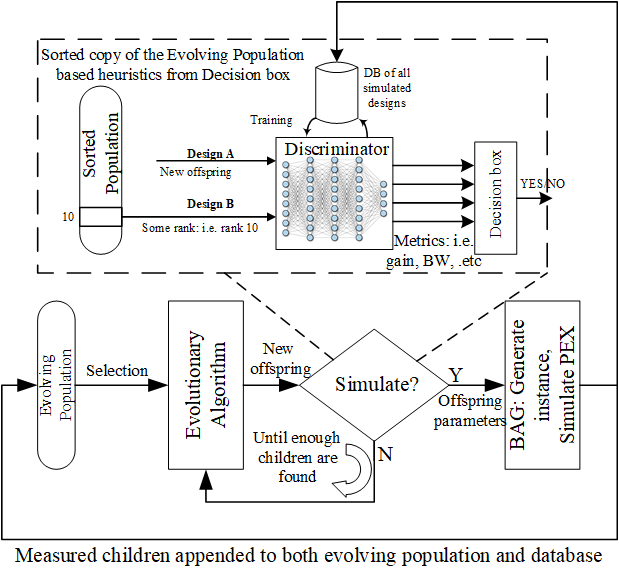Let’s describe the figure above. Our algorithm uses some underlying
evolutionary algorithm that has a broad exploration strategy. We used deap to
implement our algorithm’s evolutionary strategies (code found here).

Our algorithm starts by randomly sampling the design space (for let’s say 100
designs) and simulates all of them (this part takes some time). Then the
evolutionary algorithm proposes some offspring for future generations. We then
use the predictive model to “guess” whether the new proposed offspring is
better than some average design in the current population. So that when we
really simulate, it doesn’t get eliminated.

We should keep re-training the model as more samples are added to our database;
otherwise, the model gets biased to a specific region and we lose accuracy as
we progress. DAgger does this for imitation learning, however the big
difference here is that we can’t really re-label (simulate) rejected samples as
it defeats the purpose of short run time, so we only simulate those samples
which get approved by the model (either by mistake or correctly).

What’s that decision box at the output of the discriminator? That’s basically
telling the prediction process, the criteria to be used for discrimination.
Remember that output of model is whether a design is better than some other
design in individual metrics (not overall). The decision box in summary, keeps
track of the metrics we improved so far and the collection of metrics that
the paper, and the code.

Finally, Experiments!

We tested this methodology on a couple of circuits with different settings,
gradually making the complexity more similar to today’s analog circuit design
problems. The simplest relevant scenario is design of an opamp, verified in
schematic (with no layout) so that simulation is cheap and we can run an oracle
discriminator (based on real simulations rather than prediction of the model).

We compared that to our approach and the normal evolutionary algorithm. The
details of the circuit are in the paper, but let’s look at the performance
curve to prove a point here.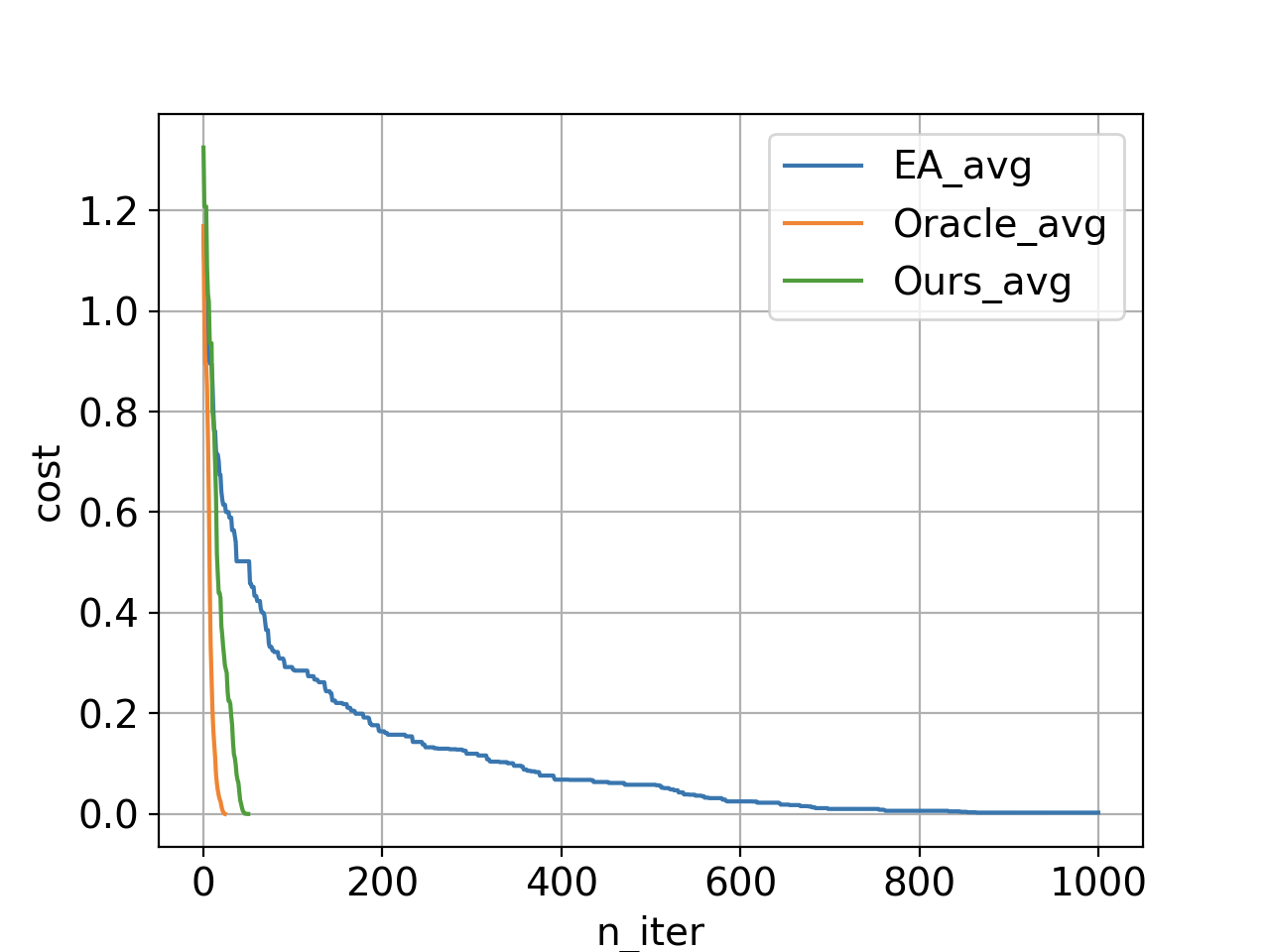In this plot we are looking at the average cost function of the top 20
individuals in the current population as a function of number of iterations. In
each iteration we simulate 5 designs and evict the worst 5 designs (to keep the
evolving population size constant). If we use just the underlying evolutionary
algorithm (without any discriminations) we get the blue curve (with over 5000
simulations); however, our method with neural network discrimination produces
the green curve (with only 240 simulations). The orange curve shows the same
method if we use the simulator the do the discriminations. Conducting the
orange experiment requires simulation of all proposed samples and keeping top 5
that are actually better than design rank 20 in the current population, which
means a lot of simulations (3400 simulations). The gap between the orange and
green illustrates how loss of accuracy due to utilization of function
approximators affects the overall optimization performance. Reaching zero means
we have at least 20 designs satisfying all the specs.

We also experimented with an optical photonic receiver design – a circuit of
bigger size and longer simulations – to see if this method can be applied to
long post-layout simulations of designs which are more complex. In this
particular example we also showed that we can even design circuits with very high
level specifications. For this example the search space was of size \$2.8 times
10^{30}\$ and to find the optimal designs we queried the discriminator 77487
times from which we only ran 435 simulations. This means that if we had to
simulate all of them we had to wait 300x longer.

Future Directions

Evaluating the performance of the algorithm in a quantitative way is still a
challenging problem. For the simple example above, we used comparisons
against an oracle. However, as our circuits get bigger and more complex,
running the oracle as a baseline quickly becomes unfeasible. How much certain
choices in the architecture affected the total performance is an open question.
We are looking into various figures of merit to account for both diversity and
quality of approved samples and compare models with different choices using
that.

In the results presented, we used full-accuracy post-layout simulations to
optimize the system. These alterations to the circuit sizing reflected both
fundamental circuit tradeoffs, as well as effects due to layout parasitics.
Because schematic simulation is significantly faster than post-layout
simulation, one direction for this work is to attempt to learn the circuit
tradeoffs from the schematic level simulation, and then refine the models based
on post-layout data.

We refer the reader to the following paper for details:

Days
:
Hours
:
Minutes
:
Seconds

Plug yourself into AI and don't miss a beat

Toronto AI is a social and collaborative hub to unite AI innovators of Toronto and surrounding areas. We explore AI technologies in digital art and music, healthcare, marketing, fintech, vr, robotics and more. Toronto AI was founded by Dave MacDonald and Patrick O'Mara.